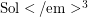# Which compact boundaryless 3-manifolds embed smoothly in the 4-sphere?

 Importance: High ✭✭✭
 Author(s): Kirby
 Subject: Topology
 Keywords: 3-manifold 4-sphere embedding
 Posted by: rybu on: November 7th, 2009
Problem   Determine a computable set of invariants that allow one to determine, given a compact boundaryless 3-manifold, whether or not it embeds smoothly in the 4-sphere. This should include a constructive procedure to find an embedding if the manifold is embeddable.

For general 3-manifolds this problem is fairly wide-open. But for some specific families of 3-manifolds it is heavily investigated.

There are two common embedding constructions: (1) obtain your 3-manifold as 0-surgery on a link which is the disjoint union of two smooth slice links. (2) Obtain your 3-manifold as the boundary of a Mazur manifold -- where Mazur manifold is taken to be a contractible 4-manifold constructed asunion a 2-handle. In both cases the resulting 3-manifold M embeds smoothly in. There are many other embedding constructions but no known "uniform" construction that works for all embeddable 3-manifolds.

Since such a 3-manifold would bound two 4-manifolds on either side, the embedding problem is a type of double cobordism problem, and related to issues such as the problem of determining which homology 3-spheres bound homology 4-balls.

The smoothness in the assumption is important. Mike Freedman has proven all homology 3-spheres admit tame topological embeddings into. These embeddings have a less combinatorial nature than smooth embeddings so it is somewhat natural to restrict to the question of smooth embeddings. For example, the Poincare Homology Sphere does not embed smoothly in, since it has a non-trivial Rochlin invariant.

## Bibliography

[B] R. Budney, Embeddings of 3-manifolds in the 4-sphere from the point of view of the-tetrahedron census, arXiv preprint arXiv:0810.2346

[CH] J.S. Crisp, J.A. Hillman, Embedding Seifert fibred-manifolds and-manifolds in-space,} Proc. London Math Soc. (3) (1998), no. {\bf 3} 685--710.

[KK] A.~Kawauchi, S.~Kojima, Algebraic classification of linking pairings on-manifolds, Math. Ann. {\bf 253} (1980), no. 1, 29--42.

[FS] R.~Fintushel, R.~Stern, Rational homology cobordisms of spherical space forms, Topology, {\bf 26} no. 3 pp. 385--393, (1987).

[GL] P.M.~Gilmer, C.~Livingston, On embedding 3-manifolds in 4-space, Topology, {\bf 22}, no. 3, pp. 241--252 (1983).

*[K] Kirby, R. Problem list in low-dimensional topology. [http://math.berkeley.edu/~kirby/problems.ps.gz]

[L] R.A.~Litherland, Deforming twist-spun knots, Trans. Amer. Math. Soc. {\bf 250} (1979), 311--331.

* indicates original appearance(s) of problem.